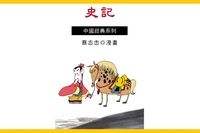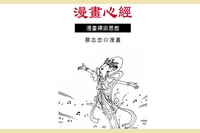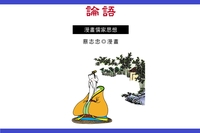菜根譚

• 點閱：88
• 作者：
• 出版年：2007[民96]
• 出版社：蔡志忠
• 出版地：臺北市
• 集叢名：中國漫畫經典-中國經典系列章節

• 淡中知真味(p.1)
• 忙裏悠閑(p.7)
• 澹泊明志(p.13)
• 眼前放得寬大(p.21)
• 路要讓一步(p.27)
• 俠義交友(p.33)
• 退即是進(p.37)
• 驕矜無功(p.43)
• 動靜合宜(p.49)
• 山林士胸懷廊廟(p.56)
• 做事勿太苦(p.62)
• 知退一步之法(p.68)
• 待小人不惡(p.75)
• 超越天地之外(p.81)
• 修得須忘功名(p.88)
• 真偽之道(p.94)
• 忘功不忘過(p.100)
• 惡人讀書(p.106)
• 讀書希聖講學躬行(p.112)
• 苦中有樂(p.119)
• 謙虛受益(p.125)
• 勿羨貴顯(p.131)
• 為惡而畏人知(p.137)
• 泛駕之馬可就馳驅(p.144)
• 人只一貪念私(p.151)
• 耳目見聞為外賊(p.160)
• 圖未就之功(p.167)
• 風來疏竹(p.173)
• 靜中靜非真靜(p.180)
• 平民肯種德施惠(p.186)
• 文章做到極處(p.192)
• 天地有萬古(p.198)
• 老來疾病(p.204)
• 小處不滲漏(p.210)
• 千金難結一時之歡(p.216)
• 遇沉沉不語之士(p.222)
• 覺人之詐(p.226)
• 吾身一小天地也(p.231)
• 父慈子孝(p.237)
• 有妍必有醜為之對(p.246)
• 德者才之主(p.253)
• 鋤奸杜幸(p.258)
• 饑則附(p.266)
• 君子宜拭冷眼(p.272)
• 一燈螢然(p.279)
• 魚網之設(p.286)
• 水不波則自定(p.292)
• 謝事當謝於正之盛時(p.299)
• 交市不如友山翁(p.303)
• 德者事業之基(p.309)
• 心者後裔之根(p.313)
• 拋卻自家無盡藏(p.317)
• 道是一重公眾事(p.323)
• 人之過誤宜恕(p.329)
• 我貴而人奉之(p.335)
• 為鼠常留飯(p.341)
• 居官有二語(p.347)
• 處富貴之地(p.353)
• 休與小人仇讎(p.357)
• 毋憂拂意(p.363)
• 飲宴之樂多(p.369)
• 冷眼觀人(p.374)
• 性躁心粗者(p.380)
• 聞惡不可就惡(p.386)
• 用人不宜刻(p.392)
• 不可乘喜而輕諾(p.398)
• 口乃心之門(p.404)
• 君子處患難而不憂(p.412)
• 談山林之樂者(p.418)
• 釣水(p.424)
• 歲月本長(p.433)
• 得趣不在多(p.441)
• 聽靜夜之鐘聲(p.448)
• 人解讀有字書(p.452)
• 心無物欲(p.459)
• 會得個中趣(p.465)
• 山河大地已屬微塵(p.470)
• 石火光中爭長競短(p.477)
• 延促由於一念(p.483)
• 松澗邊(p.489)
• 隱逸林中無榮辱(p.495)
• 矜名不若逃名趣(p.499)
• 嗜寂者(p.505)
• 孤雲出岫(p.511)
• 饑來吃飯倦來眠(p.515)
• 水流而境無聲(p.523)
• 山林是勝地(p.529)
• 蘆花被下(p.535)
• 忽聞犬吠雞鳴(p.541)
• 我不希榮(p.545)
• 徜徉於山泉石之間(p.550)
• 春日氣象繁華(p.556)
• 一字不識(p.561)
• 身如不系之舟(p.571)
• 欲其中者(p.576)
• 多藏者厚亡(p.582)
• 竹影掃階塵不動(p.588)
• 魚得水逝(p.595)
• 狐眠敗兔(p.601)
• 寵辱不驚(p.607)
• 晴空朗月(p.615)
• 才就筏便思舍筏(p.622)
• 詩思在灞陵橋上(p.626)
• 真空不空(p.632)
• 飽諳世味(p.643)
• 性天澄徹(p.649)
• 金自礦出(p.655)
• 神酣布被窩中(p.661)
• 萬慮都捐(p.667)
• 不如放身心(p.674)
• 當雪夜月天(p.681)
• 優人傅粉調朱(p.687)
• 人生福境禍區(p.693)
• 繩鋸木斷(p.699)
• 雨餘觀山色(p.705)
• 登高使人心曠(p.709)
• 心曠心隘(p.715)
• 就一身了一身者(p.723)
• 人生太閑(p.729)
• 耳根似飆穀投響(p.735)
• 花看半開(p.741)
• 人生原是一傀儡(p.748)
• 茶不求精而壺亦不燥(p.753)
• I D E A 大公開(p.761)同作者書籍史記漫畫心經:漫畫禪宗思...論語:漫畫儒家思想